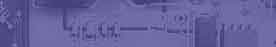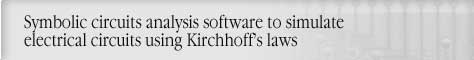Phasor Diagram & Complex Numbers for AC Circuits AnalysisCircuit analysis Tutorial
AKNM Circuit Magic- circuit analysis software

Phasor Diagram & Complex Numbers for AC Circuits Analysis

As we saw above, sinusoidal waveform can be defined by three variables (Frequency, Magnitude, Phase). In case then all sources frequency are equal, sinusoidal waveform can be defined by two variables (Magnitude, Phase). Phase and Magnitude defines a phasor(vector) or complex number in polar form. Click here to learn about complex numbers.. In this case? magnitude is Vector lenght, phase is the angle betwen phasor & x axis.

To determine instantaneous value at the same time, we rotate vector on angle corresponding this time. Projection to Y (j) axis are instantaneous value.

On applet below enter magnitude, phase then press draw button. Applet draws phasor and calculates real & imaginary part of complex. Press start button to run simulation.